# Dilations, Scale Factors, and Scale Drawings Lesson Plan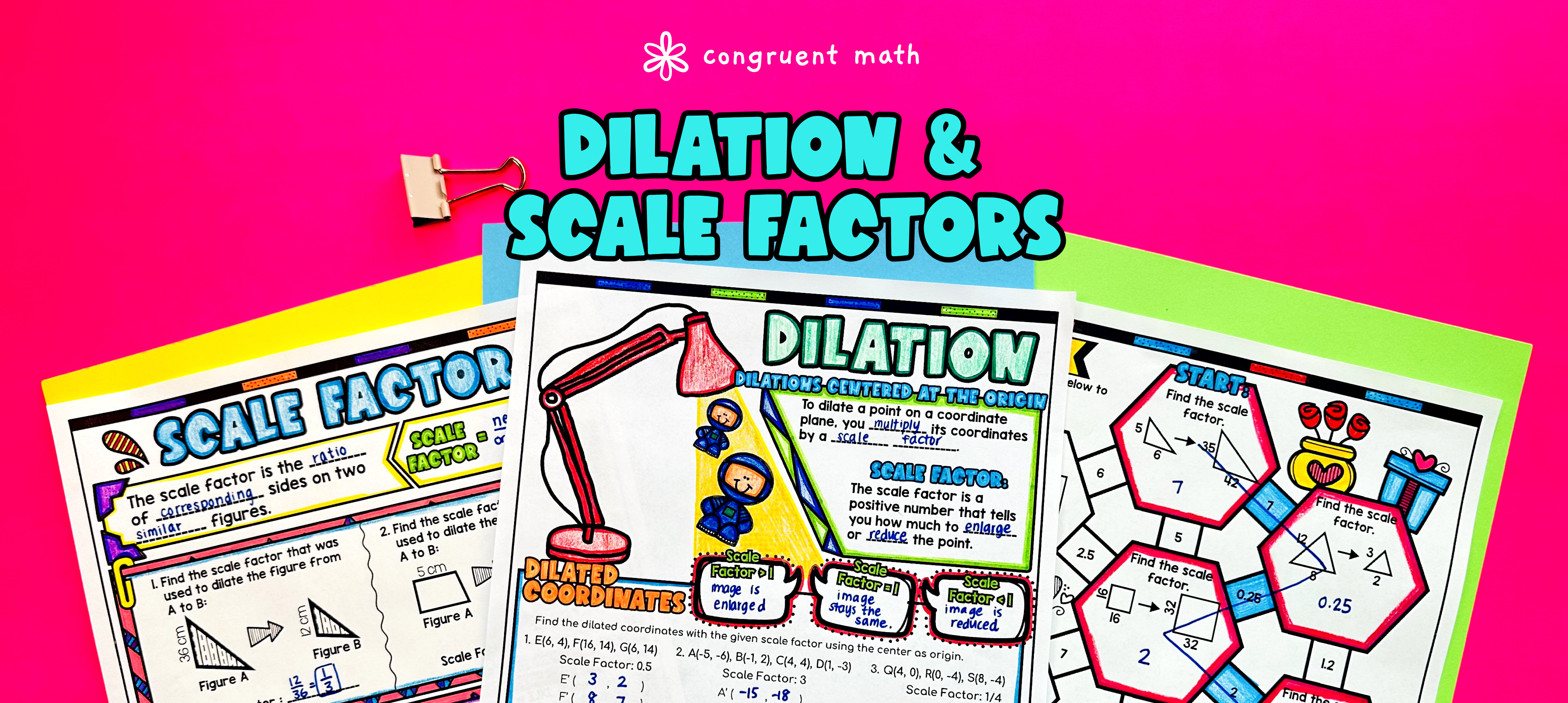Pin This

## Overview

Ever wondered how to teach dilations, scale factors, and scale drawings in an engaging way to your 7th and 8th grade math students?

In this lesson plan, students will learn about dilations, scale factors, scale drawings, and their real-life applications. Through artistic and interactive guided notes, check for understanding, practice worksheets, and self-checking pixel art activities, students will gain a comprehensive understanding of these mathematical concepts.

The lesson begins with guided notes that introduce the concepts of dilatations, scale factors, scale drawings, and similar figures. The notes include embedded checks for understanding, practice worksheets to reinforce the concepts, and a real-life application of math.

Following the guided notes, students will engage in self-checking pixel art activities on Google Sheets. These activities provide immediate feedback, with incorrect answers staying red and correct answers turning green to reveal a mystery picture. This interactive and creative approach helps students practice dilations, scale factors, and scale drawings while having fun.

The lesson culminates with a real-life example that explores how dilations and scale drawings are applied in the real world. This application helps students see the relevance and practicality of the mathematical concepts they have learned.

By the end of this lesson plan, students will have a solid understanding of dilations, scale factors, scale drawings, and similar figures. They will be able to apply these concepts to solve problems and recognize their importance in various real-life situations.

## Get the Lesson Materials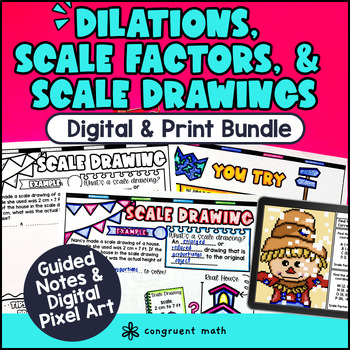\$10.99

## Learning Objectives

After this lesson, students will be able to:

• Define dilations, scale factors, and scale drawings

• Identify and label similar figures

• Calculate scale factors using ratios

• Create scale drawings using given scale factors

• Explain how dilations, scale factors, and scale drawings are used in real life

## Prerequisites

Before this lesson, students should be familiar with:

• Basic geometric concepts such as graphing on coordinate planes

• Knowledge of basic multiplication and division skills

• Basic understanding of fractions and decimals (optional, but helpful)

## Key Vocabulary

• Dilations

• Scale factors

• Scale drawings

• Similar figures

• Transformations

## Procedure

### Introduction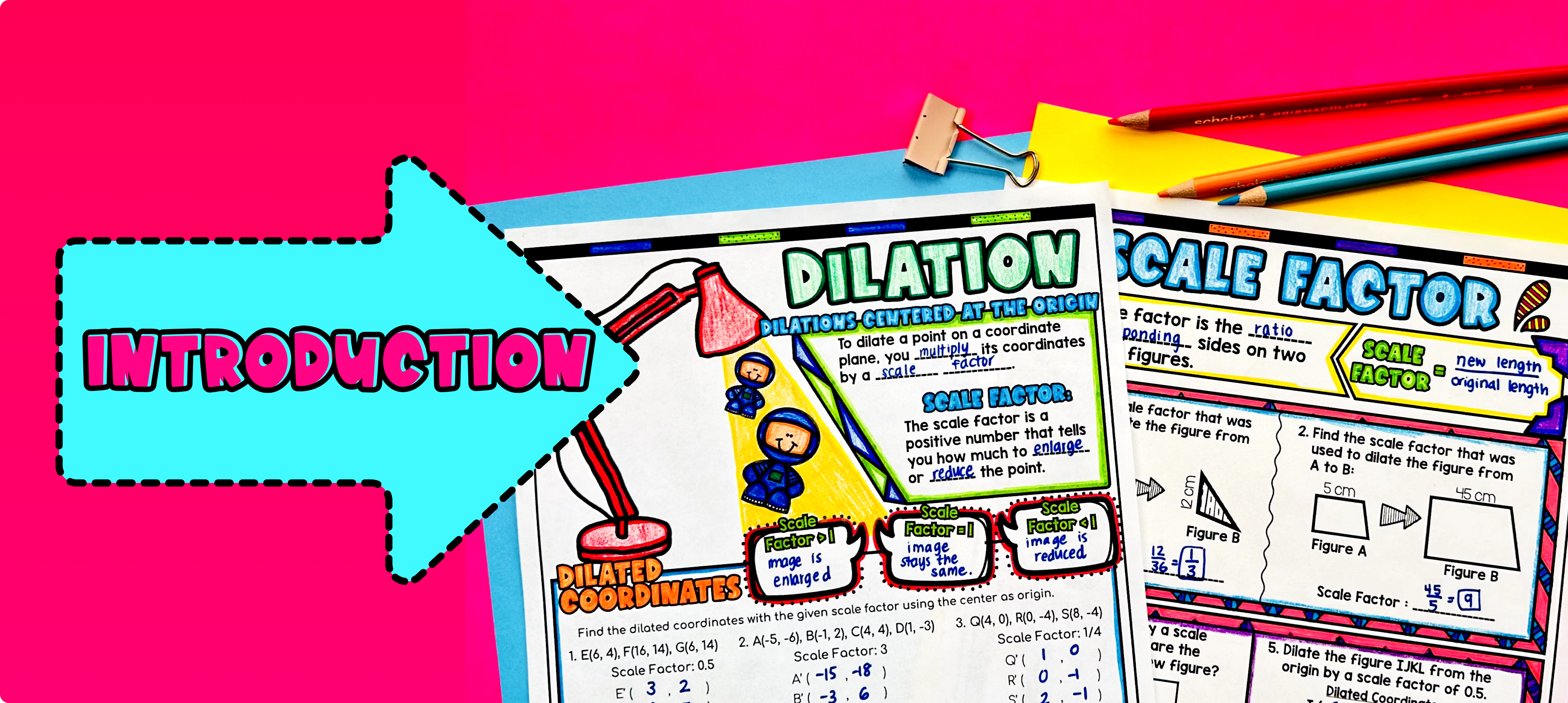Pin This

As a hook, ask students why understanding dilations, scale factors, and scale drawings is important in real life. Refer to the last page of the guided notes for ideas.

Use the first page of the guided notes to introduce dilations and scale factors. Walk through the key points of these topics to teach, such as explaining what dilation is and how scale factors relate to dilations. Then, have students fill in the guided notes portion stating what happens when the scale factor is less than 1 (reduction), equal to 1 (no change) and more than 1 (enlargement). Allow students to color & have some fun as they take notes!

Then, move to the bottom portion of the first page of the guided notes and model how to find dilated coordinates, as well as graphing dilated figures. Based on student responses, reteach concepts that students need extra help with. If your class has a wide range of proficiency levels, you can pull out students for reteaching. Have students fill out the fun fact portion of the notes.

Then, use page 2 of the guided notes to teach scale factors. Model one example of how to calculate scale factors and then have students try the rest.

### Practice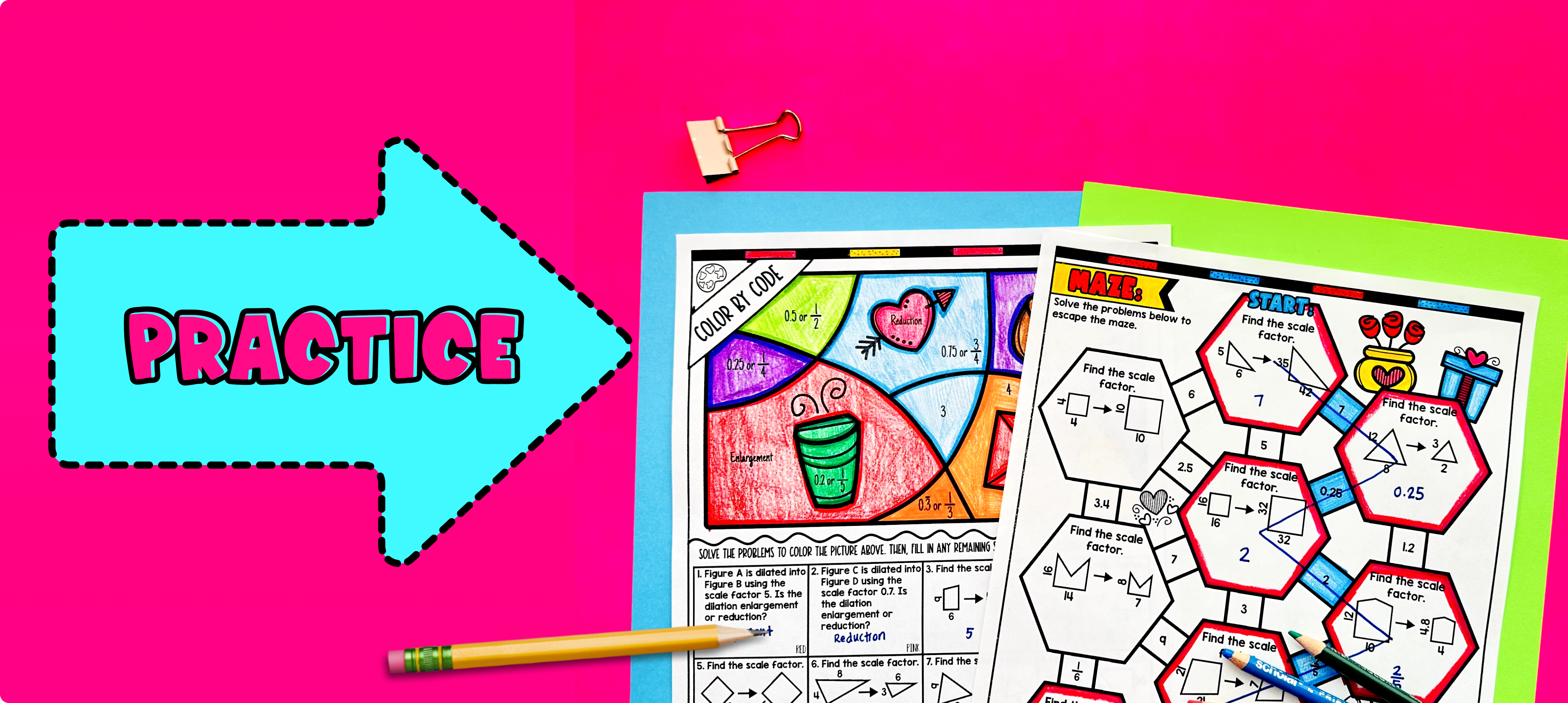Pin This

Have students practice dilations, scale factors, scale drawings, and similar figures using pages 3-4 of the guided notes. There is an included color by number and maze activity to make the practice fun! Walk around the classroom to answer any student questions and provide guidance as needed.

### Real-Life Application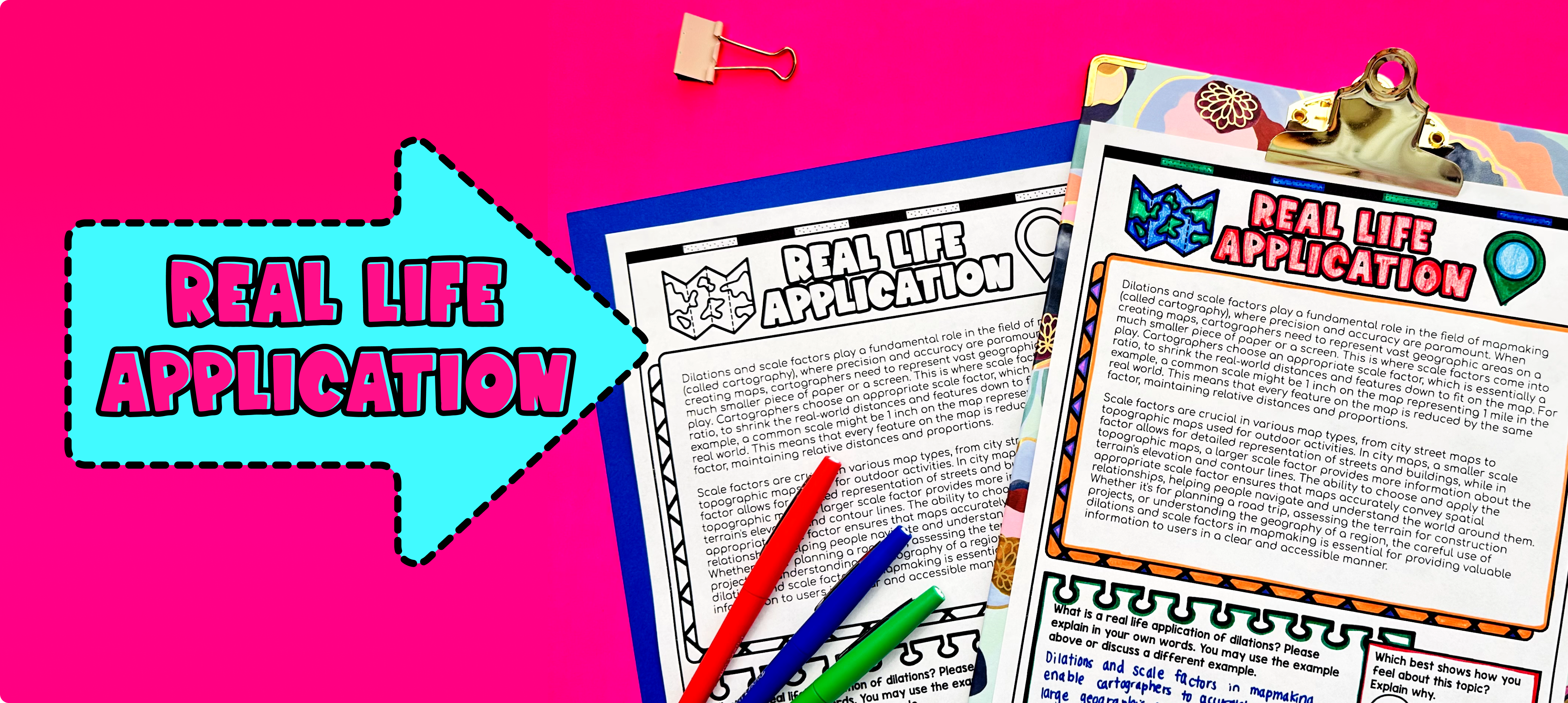Pin This

Bring the class back together, and introduce the concept of real-life applications of dilations, scale factors, and scale drawings. Explain to the students that these mathematical concepts have practical uses in various fields, such as architecture, engineering, and graphic design. Use the last page of the guided notes to have students read about how scale factors is used in mapmaking.

Ask the students to brainstorm different real-world scenarios where dilations, scale factors, and scale drawings are used. Encourage them to think about situations where it is necessary to change the size of an object or image while maintaining its proportions. Some examples could include:

• Architectural blueprints: Architects use scale drawings to represent large buildings on a smaller scale. By using a scale factor, they can create detailed plans that accurately represent the different components and measurements of the building.

• Maps: Cartographers use dilations and scale factors to create maps that represent large areas on a smaller scale. This allows people to navigate different areas and understand the relative distances between locations.

• Engineering designs: Engineers use dilations and scale factors to design and construct various structures, such as bridges and skyscrapers. By creating scaled models, engineers can test the structural integrity and ensure that the final product will be safe and functional.

Refer to the FAQ section on the teaching resource for more ideas on how to teach real-life applications of dilations, scale factors, and scale drawings.

## Extensions

If you’re looking for digital practice for dilations, scale factors, and scale drawings, try my Pixel Art activities in Google Sheets. Every answer is automatically checked, and correct answers unlock parts of a mystery picture. It’s incredibly fun, and a powerful tool for differentiation.

Here are are some activities to explore:

A fun, no-prep way to practice dilations, scale factors, and scale drawings is Doodle Math — they’re a fresh take on color by number or color by code. It includes multiple levels of practice, perfect for a review day or sub plan.

Here is an activity to try:

### Real-Life Math Project

A fun way to wrap this lesson with your students is with one of my real-life math projects. They enable students to see the application of math in an engaging, extended project. Students create scale drawings of different Minecraft characters. Minecraft is a popular video game in middle school!

This activity is NOT AN OFFICIAL MINECRAFT PRODUCT. NOT APPROVED BY OR ASSOCIATED WITH MOJANG.

## Want more ideas and freebies?

Get my free resource library with digital & print activities—plus tips over email.